• ### Miller's Pitch Shifting Example From His Book

I've never really dug into pitch shifting before and I have started looking at Miller's pitch shifting patch example from his book but I'm a little confused by the graph in this related example, specifically Fig 7. 17. (I think just because how the qualifying text is worded). I have a few clarifying questions so hopefully someone can humor me.1. The diagonal line from origin (0,0) is the input signal; the dotted line is the variable delay line; the diagonal line at D is the delay line over time relative to the input signal, yes?
2. D is the distance from the origin point to the point marked D on the x axis, correct? This is the max length of the delay line?
3. The y axis shows the quantity `n - d[n]` where `d[n]` represents the delay in samples and this is where I get a bit fuzzy: graphically, what is the output sample here? Why am I subtracting? What am I subtracting?

• Posts 21 | Views 6455
• @ricky Finally! Someone else who's been studying this book!Think of the x and y axis as the index of the output and input samples respectively. So if you're not delaying at all, then at output time 42 the delay line will output the input sample at time 42. That's what's expressed by the diagonal line from the origin. Everything above that line would be impossible without a crystal ball: for instance at output time 10 you can't output the input sample at time 50--that would be looking 40 time units into the future!

You're right about D being the maximum delay line length, but I think of it as the horizontal distance between those diagonal lines because that maximum length applies at all times. Everything below the diagonal from D would be impossible because the delay line can't store input samples more than D time units old.

So what you're subtracting are sample indexes, not the samples themselves. All that formula is saying is that at any given output time n, the delay line is outputting an earlier input sample, earlier by d[n]. Does any of this help?

Here's one more observation about this graph that might help clarify it: if one were graphing a fixed 10ms delay, it would be a line parallel to the origin diagonal, but 10ms to the right of it. With that, you can see that the dotted line starts at some delay amount, lengthens as output time progresses, then stops at some greater delay amount.

• @jameslo, Thanks! It is super helpful to talk through it with someone else. My understanding is aligned with yours. I am relievedThe parallel line description is precisely where I had arrived when reading through this material, so thanks for confirming. I have some follow-ups so hopefully you're game to keep the conversation going. I am looking at the pitch shifting page.

This graphic is a bit confusing to me, too. Or at least I'm not sure if I am reading it correctly.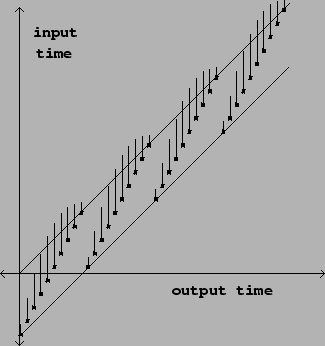How does the vertical line above each point actually relate to enveloping? Additionally, I am unsure how their trajectory actually results in a continuous pitch shift. In this illustration, I think the sample points are representative of a decreasing delay line time over each period and if there is continuity between each ascending sample (which would pitch shift our input signal up, as I understand it?) this would lead to a continuous pitch shift?

I'm trying to understand more clearly how these graphics actually relate to the idea of Momentary Transposition as it's described in the book.

• @jameslo - actually one small follow-up from your comment about the index via `n-d[n]`. I guess I am not sure how that would be read graphically. I get that we arrive at a new index value through subtraction but where does `n` intersect on this graph? Where does `d[n]` intersect? Hopefully that question makes sense. It might help to plug in some values.

• @ricky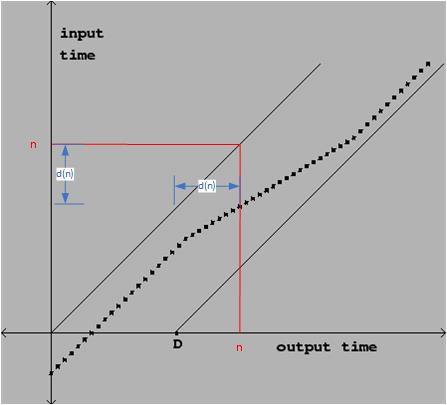n is the given output sample index. You go up from there to intersect the origin diagonal as well as the one dot. The dot is d(n) to the right of the diagonal. Therefore, you want to output the input sample at n-d(n)

• Ah! So `d[n]` is basically the distance between the original input signal (no delay) and the variable delay.

So the output sample here would be at the origin diagonal at the bottom of the blue vertical line as you've drawn it?

Would I be correct in saying that the variable delay line is pitching down at that intersection in the illustration you've modified?I'd still love to know if when sample points in the diagram above are representative of a decreasing delay line time over each period and if there is continuity between each ascending sample (which would pitch shift our input signal up, as I understand it?) this would lead to a continuous pitch shift? My intuition says yes but it's fun to talk about these things, I think.

I guess that's what Miller means by, "If `d[n]` does not change with n, the transposition factor is 1 and the sound emerges from the delay line at the same speed as it went in. But if the delay time is increasing as a function of n, the resulting sound is transposed downward, and if d[n] decreases, upward."

• @ricky

So the output sample here would be at the origin diagonal at the bottom of the blue vertical line as you've drawn it?

yes

Would I be correct in saying that the variable delay line is pitching down at that intersection in the illustration you've modified?

yesThe vertical lines are confusing, but he's trying to show how each of the input samples is weighted in the window envelope. Compare with the dataflow diagram fig 7.21.

• Cool. Thanks, @jameslo.

So, getting to the Pitch Shifter example itself, it's easy enough to follow the transposition factor from the math to patch but I'm wondering why the -1 preceding the tape head rotation frequency isn't the current sample rate, as per the math?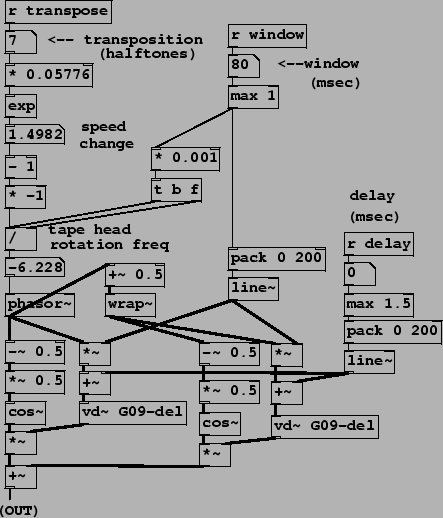`f = (t - 1) * R / s`

I see where one is subtracted from t and where the window size is divided into that but why does R = -1? Is this some other interpretation of 'sample rate?'

• @ricky Having looked for 10 minutes I can't figure out how the formula for f relates to the computation in the sample patch, but just looking at the patch itself, the negative frequency (i.e. multiplication by -1) makes sense to me because you want ever decreasing delay amounts. [phasor~] is an increasing ramp with positive frequencies.

• So, you're saying the `-1` is in place to ensure we get decreasing delay amounts for when we want to pitch up and increasing delay amounts when we want to pitch down because of the nature of phasor~? That makes sense but it doesn't explain the `R` in the math.

• @jameslo - I remembered this live granular synthesis example from the Pd tutorial website which makes sense to me.

The transposition factor is handled as `pow(2, t/12) - 1 * 44100/s)` where t is the transposition step and s is the windows size. Maybe we can reconcile the differences and figure out how Miller factor the sample rate.

• It's a bad idea to post after cocktail hour, but here goes:

Regarding sample rate R, go back to the original transposition formula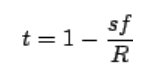Note that R is in samples/second and s is in samples/window. But in the example patch, s is specified in seconds (after the multiplcation by 0.001). So that factors out the sample rate coming from the transposition factor because you'd have to multiply s in seconds by the sample rate to get s in samples.

But that doesn't explain the [* -1]. I think there's just an algebra mistake in the text. Go ahead and solve the original transposition formula for f and you'll see that the sign is flipped.

• Cocktail hour is the best hour. Good man and good catch! I totally forgot about the 0.001 multiplier and of course it factors it out. D'oh. As for the [* -1], I thought this made sense in the context of the Pd patch given the positive ramp of the phasor and without it the pitch transposition step is inverted.

What you're saying is that the algebra below is incorrect? Maybe it has something to do with the way the transposition factor is calculated?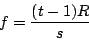It's too early for a cocktail here. Still on my first coffee.

• I'm serious: solve the original transposition formula for f. Show your work• @ricky It's [- 1] because the exponent of 0 (no shift) is 1 but the tape head has to be stationary (no rotation = 0) for playback at normal speed......
And [* -1] because (although it seems wrong until you really think about it) the tape head has to be turning backwards relative to the tape (negative values for [phasor~]...) for the pitch to increase (positive transposition values).
There is no point arguing with the patch because it works as expected.......[cos~] windows the output so that amplitude sums are "sort of" ok (with the in and out of phase bits) and the vertical edge of the [phasor~] saw is declicked.
And of course the change of delay gives the rotating read head effect.

@katjav gave it a lot more thought here........ https://www.katjaas.nl/pitchshift/pitchshift.html
I could be wrong but I don't see why the samplerate would be part of the calculation (it isn't)...... as all variables are relative.
David

• @whale-av said:

@ricky It's [- 1] because the exponent of 0 (no shift) is 1 but the tape head has to be stationary (no rotation = 0) for playback at normal speed......

Ah, thanks. That makes sense. Thanks, David.

And [* -1] because (although it seems wrong until you really think about it) the tape head has to be turning backwards relative to the tape (negative values for [phasor~]...) for the pitch to increase (positive transposition values).
There is no point arguing with the patch because it works as expected.......Who is arguing?This was my understanding.

[cos~] windows the output so that amplitude sums are "sort of" ok (with the in and out of phase bits) and the vertical edge of the [phasor~] saw is declicked.

Yes, a nicely scaled fade in and out.

• @whale-av said:

@katjav gave it a lot more thought here........ https://www.katjaas.nl/pitchshift/pitchshift.html
I could be wrong but I don't see why the samplerate would be part of the calculation (it isn't)...... as all variables are relative.

I was confused because R represents sample rate earlier in the text.

"If the frequency of the sawtooth wave is \$f\$ (in cycles per second), then its value sweeps from 0 to 1 every \$R/f\$ samples (where \$R\$ is the sample rate)."

• @ricky I think that is just to work out a good window size with enough samples in it but not too many,
The relationship between a semitone interval and frequency is a ratio....... discovered long before digital audio when "samplerate" might have referred to wine tasting (a bit before cocktails too........).
As I said I could be wrong but I cannot see anything in the patch and cannot imagine how the samplerate could be relevant to the [phasor~] frequency.
Sorry about the "arguing"..... and thank you for the link. I always thought Millers book was a real paper thing, and didn't know it was online.
David.

• Hmm, it's interesting that these kinds of time-domain algorithms use cosine-shaped windows and 2X overlap, whereas FFT resynthesis algorithms use Hann windows and 4X overlap. I've fooled around a little with FFT resynthesis using the former, and the sound is just a little coarser, which is to say I was surprised it wasn't terrible. I wonder if for something like time-domain pitch shift Hann+4X overlap would sound better (i.e. have softer windowing artifact) and if so, why.

(This question occurs to me just as I'm preparing to have no free time for a week so that's why I'm not just trying it and posting my results with further questions)

• I thought the cosine shape was really just convenience given that [cos~] is available and that allows for a sample-accurate solution that doesn't involve reading from a table.

• For future students & fellow nerds, here’s what I was suggesting: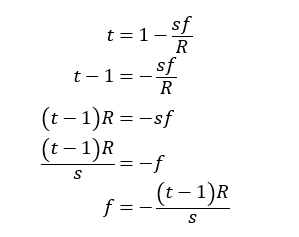See? Opposite sign, contrary to the text.

RE sample rate, let s’ = s/R (in English: specify the window size in seconds instead of samples). Then: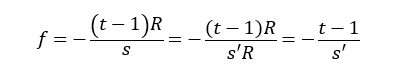which is what the example patch G09 is computing for the phasor frequency.

RE Hann windowing with 4x overlap, it definitely sounds worse in this case, still not sure why when it's better for the FFT.
time domain overlapped windowing.zip
I have a faint memory of an explanation in Miller's book why the positive part of the cosine function is a useful windowing function for time domain stuff, but I can't find it. Maybe I just saw it used a lot in the audio examples.

Posts 21 | Views 6455
Internal error.

Oops! Looks like something went wrong!